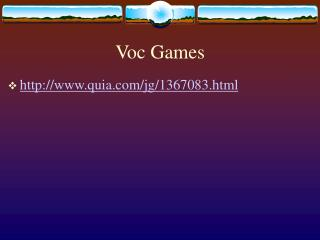DownloadDownload PresentationVoc Games

# Voc Games

Télécharger la présentation## Voc Games

- - - - - - - - - - - - - - - - - - - - - - - - - - - E N D - - - - - - - - - - - - - - - - - - - - - - - - - - -
##### Presentation Transcript

1. Voc Games • http://www.quia.com/jg/1367083.html

2. The English mathematician Sir Isaac Newton restated Galileo’s ideas aboutmotion in the first of his three laws of motion. Newton

3. Newton’s first law of motion states that an object at rest tends to stay at rest and an object in motion tends to stay in motion with the same speed and in the same direction unless acted upon by an unbalanced force 1st Law of Motion

4. Inertia • Newton’s first law is also called the law of inertia. An object’s resistance to a change in motion is called inertia. • The amount of inertia an object hasdepends on its mass. • Objects with greater mass have more inertia, and require a greater force to cause a change in motion.

5. Newton’s second law of motion states that acceleration depends on the object’s mass and the net force acting on the object. Acceleration = Net Force Mass 2nd Law of Motion

6. When acceleration is measured in meters per second per second (m/s2) Mass is measured in kilograms. Force is measured in kilograms × meters per second per second (kg·m/s2) Acceleration

7. Newton • This unit is called the newton (N). • One newton equals the force required to accelerate one kilogram of mass at 1 meter per second per second. • 1 N = 1 kg × 1 m/s2

8. The acceleration of an object will increase if the force increases. According to the equation, acceleration and force change in the same way—both get larger. The equation also shows that the acceleration will increase ifthe mass decreases. Acceleration and mass are inversely proportional. Acceleration and Force

9. Math Practice (calculating net force) • A speedboat pulls a 55kg water skier. The force causes the skier to accelerate at 2.0m/s2. Calculate the net force that causes this acceleration • What is the net force on a 1000 kg object accelerating at 3m/s2? • What net force is needed to accelerate a 25kg cart at 14m/s2?

10. Think about what you are trying to solve (net force) • The net force (Fnet)= • A= Fnet m Fnet = (m)(a)

11. Answers • 110N • 3000N • 350N Remember that kg x m/s2 = N